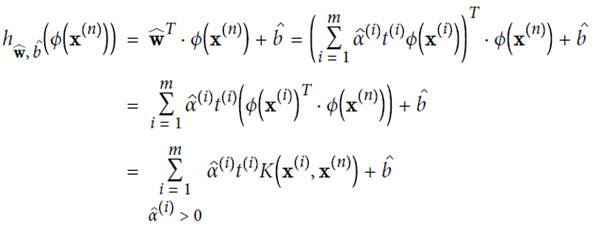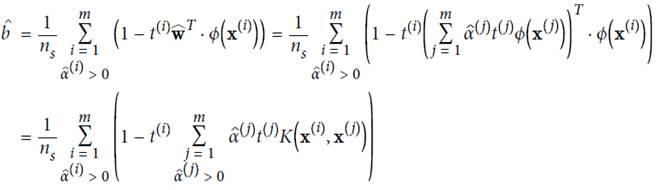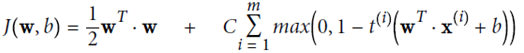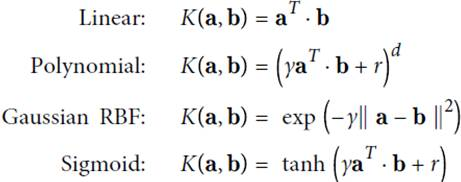# 【机器学习】支持向量机（SVM）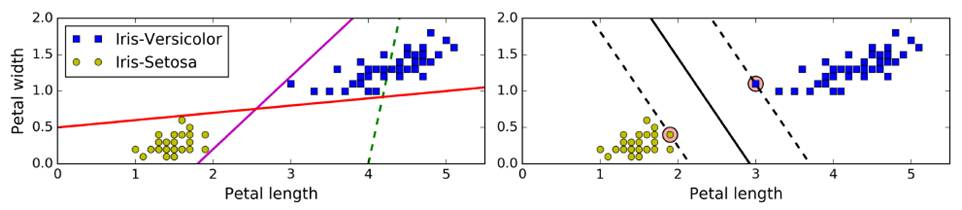SVM：拟合的不是一条线，而是两条平行线，且这两条平行线宽度尽量大，主要关注距离车道近的边缘数据点（支撑向量support vector），即large margin classification——上图右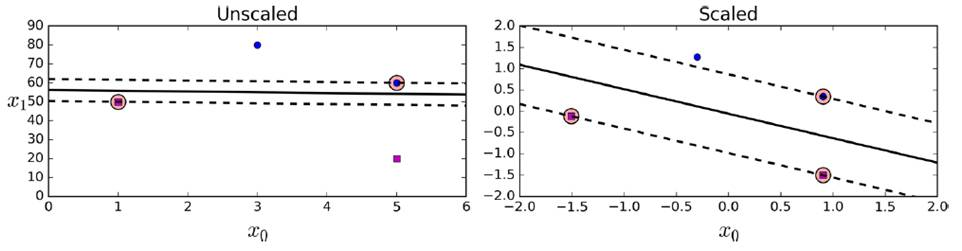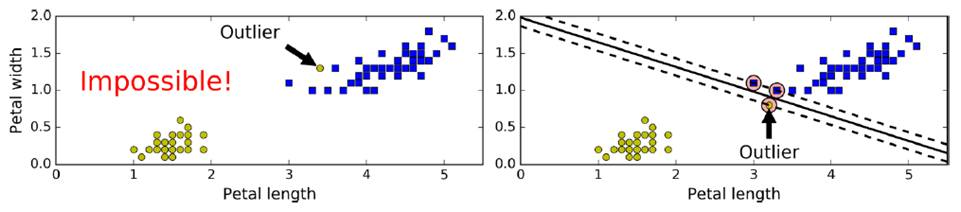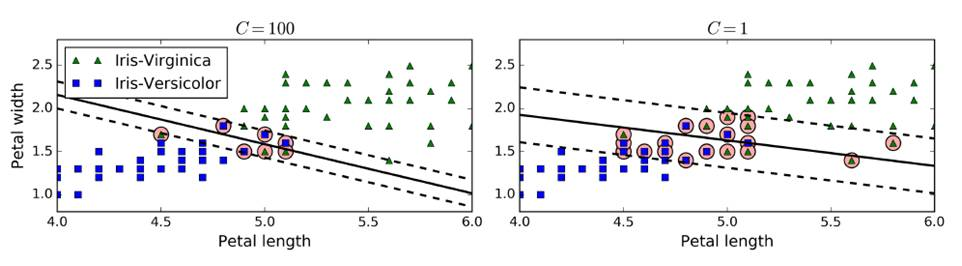SVM特有损失函数Hinge Loss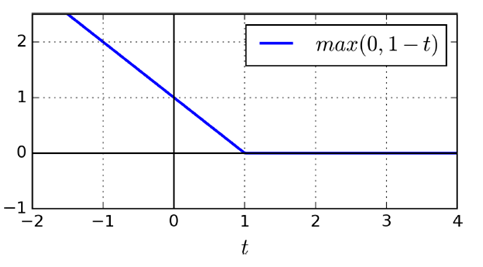LinearSVC

(liblinear库，不支持kernel函数，但是相对简单，复杂度O(m*n)）

import numpy as np
from sklearn import datasets
from sklearn.pipeline import Pipeline
from sklearn.preprocessing import StandardScaler
from sklearn.svm import LinearSVC

X = iris["data"][:,(2,3)]
y = (iris["target"]==2).astype(np.float64)
svm_clf = Pipeline((
("scaler",StandardScaler()),
("Linear_svc",LinearSVC(C=1,loss="hinge")),
))
svm_clf.fit(X,y)
print(svm_clf.predit([[5.5,1.7]]))

from sklearn.preprocessing import PolynomialFeatures
polynomial_svm_clf = Pipeline((
("poly_features", PolynomialFeatures(degree=3)),
("scaler", StandardScaler()),
("svm_clf", LinearSVC(C=10, loss="hinge"))
))
polynomial_svm_clf.fit(X, y)

SVC（基于libsvm库，支持kernel函数，但是相对复杂，不能用太大规模数据，复杂度O(m^2 *n)-O(m^3 *n)）

可以直接使用SVC（coef0：高次与低次权重）

from sklearn.svm import SVC
poly_kernel_svm_clf = Pipeline((
("scaler", StandardScaler()),
("svm_clf", SVC(kernel="poly", degree=3, coef0=1, C=5))
))
poly_kernel_svm_clf.fit(X, y)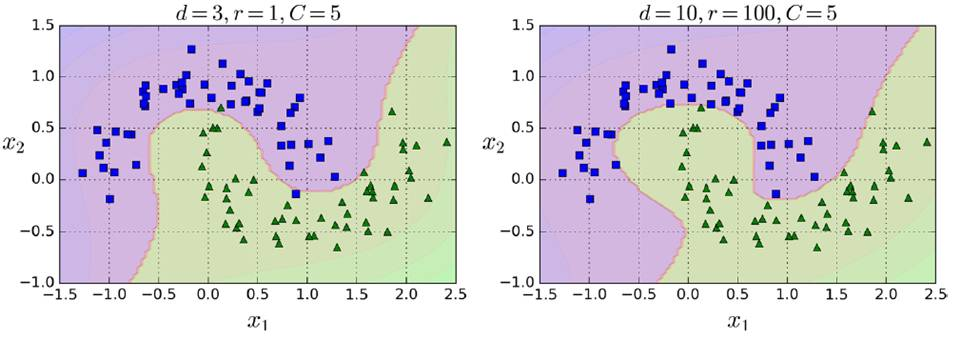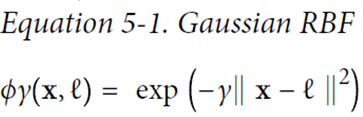gamma（γ）控制高斯曲线形状胖瘦，数据点之间的距离发挥更强作用

rbf_kernel_svm_clf = Pipeline((
("scaler", StandardScaler()),
("svm_clf", SVC(kernel="rbf", gamma=5, C=0.001))
))
rbf_kernel_svm_clf.fit(X, y)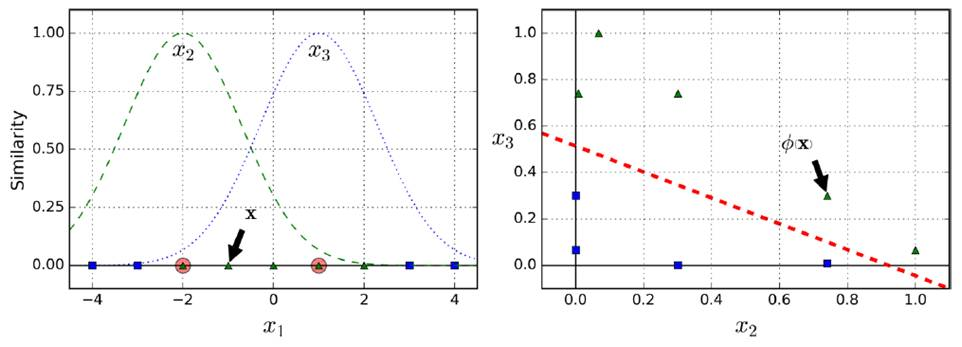SGDClassifier(支持海量数据，时间复杂度O(m*n))

SVM Regression（SVM回归）

from sklearn.svm import LinearSVR
svm_reg = LinearSVR(epsilon=1.5)
svm_reg.fit(X, y)

使用SVR

from sklearn.svm import SVR
svm_poly_reg = SVR(kernel="poly", degree=2, C=100, epsilon=0.1)
svm_poly_reg.fit(X, y)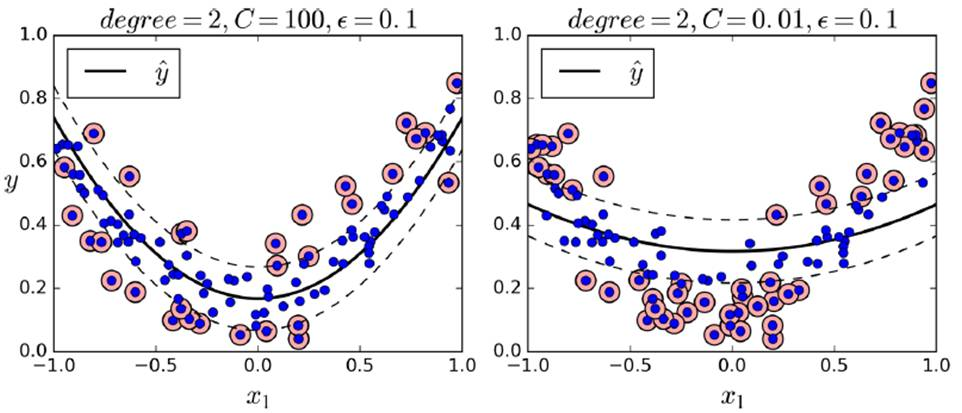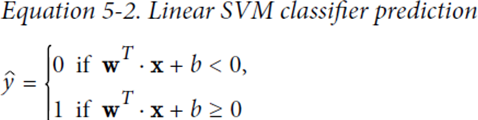w通过控制h倾斜的角度，控制车道的宽度，越小越宽，并且使得违反分类的数据点更少

hard margin linear SVM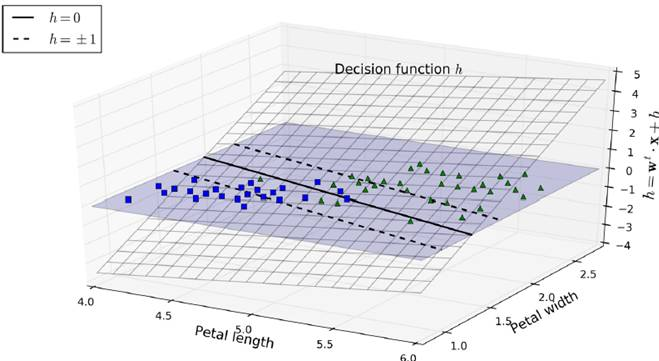soft margin linear SVM

LinearSVM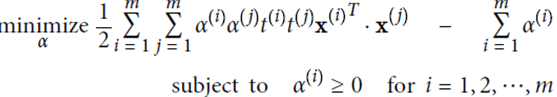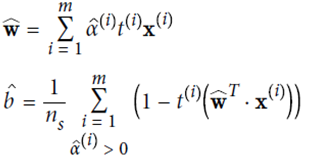KernelizedSVM